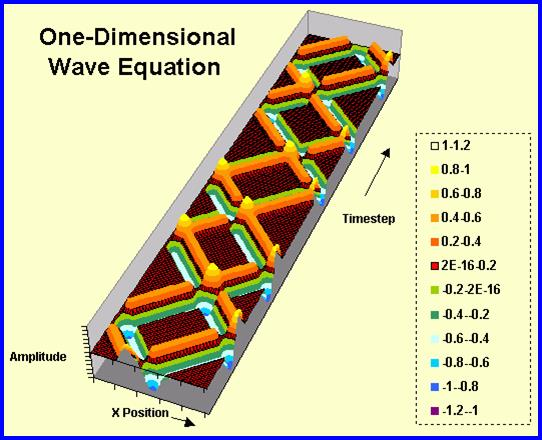# One-dimensional Wave Equation

UPDATED: 09/01/2022

This spreadsheet applies a 3-time-level differencing scheme to the one-dimensional, linear wave equation. The user can watch the time dependence of the wave as a function of spatial position or can see the complete transient as a function of space and time as seen in the contour plot below. The user can choose the Courant number to give an exact solution (c = U Δt/ Δx = 1.0), a solution demonstrating numerical dispersion (C < 1.0) or a solution demonstrating a numerical instability (C>1.0). The algorithm is directly from An Introduction to Computational Fluid Mechanics by C.Y. Chow.Numerical Solution for 1D linear wave equation in a tube. Amplitude of the wave is plotted versus x position and time. The left end of the tube is closed, while the right end is open.